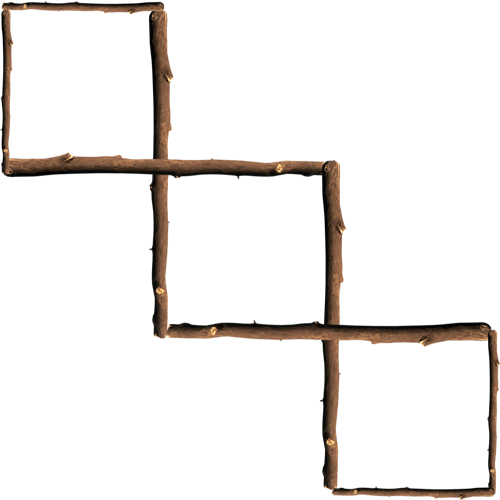Riddle: Something very extraordinary happened on the 6th of May, 1978 at 12:34 a.m. What was it?
Answer: At that moment, the time and day could be written as: 12:34, 5/6/78.
Riddle: Find the four digit number in which the first digit is one fourth of the last digit, the second digit is 6 times the first digit, and the third digit is the second digit plus 3. What is it?
Riddle: What happened to the plant in the math classroom?
Answer: It grew square roots, of course.
Riddle: What do you call two math friends?
Riddle: What is the most useful instrument in a mathematics teacher's tool kit?
Answer: Use the longer four sticks to be sharing sides between the squares and at the end their should be three intertwined squares.